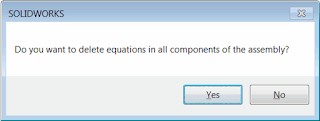# Delete all equations from SOLIDWORKS model using SOLIDWORKS API

More 'Goodies'

This macro removes all of the equations (or optionally only broken equations) in the active model (part or assembly) using SOLIDWORKS API.Equations Manager dialog

If active model is assembly, macro optionally allows to remove all equations from each component of the assembly. The following message will be displayed. Click Yes to remove equations from all components on all levels and No to only process equations of the top level assembly.Macro option to delete equations in the assembly components

Set the DELETE_BROKEN_ONLY option to True in order to only remove the broken (dangling) equations.

IMPORTANT: Use this macro on your own risk. This macro modifies your data (deletes all equations) please backup your file before running this macro

```Const DELETE_BROKEN_ONLY As Boolean = False 'if this flag is True than only broken equations are deleted, otherwise all equations are deleted

Dim swApp As SldWorks.SldWorks
Dim swModel As SldWorks.ModelDoc2
Dim swEqMgr As SldWorks.EquationMgr

Sub main()

Set swApp = Application.SldWorks

try_:
On Error GoTo catch_

Set swModel = swApp.ActiveDoc

Dim hasDeleted As Boolean

DeleteEquationsFromModel swModel, hasDeleted

If swModel.GetType = swDocumentTypes_e.swDocASSEMBLY Then

If swApp.SendMsgToUser2("Do you want to delete equations in all components of the assembly?", swMessageBoxIcon_e.swMbQuestion, swMessageBoxBtn_e.swMbYesNo) = swMessageBoxResult_e.swMbHitYes Then

Dim swAssy As SldWorks.AssemblyDoc
Set swAssy = swModel

'component needs to be loaded in memory in order to process it's equations
swAssy.ResolveAllLightWeightComponents True

Dim vComps As Variant
vComps = swAssy.GetComponents(False)

Dim i As Integer

For i = 0 To UBound(vComps)

Dim swComp As SldWorks.Component2
Set swComp = vComps(i)

Dim swCompModel As SldWorks.ModelDoc2

Set swCompModel = swComp.GetModelDoc2

If Not swCompModel Is Nothing Then

Dim hasCompEqDeleted As Boolean
DeleteEquationsFromModel swCompModel, hasCompEqDeleted

If hasCompEqDeleted Then
hasDeleted = True
End If

End If

Next

End If

End If

If hasDeleted Then
swModel.ForceRebuild3 False
End If

GoTo finally_

catch_:
swApp.SendMsgToUser2 Err.Description, swMessageBoxIcon_e.swMbStop, swMessageBoxBtn_e.swMbOk
finally_:

End Sub

Sub DeleteEquationsFromModel(model As SldWorks.ModelDoc2, ByRef hasDeleted As Boolean)

Set swEqMgr = model.GetEquationMgr()

Dim i As Integer

hasDeleted = False

'iterate in the reverse direction as the equation index will change once previous equation is deleted
For i = swEqMgr.GetCount - 1 To 0 Step -1

If Not DELETE_BROKEN_ONLY Or IsEquationBroken(swEqMgr, i) Then
swEqMgr.Delete i
hasDeleted = True
End If
Next

If hasDeleted Then
'deleting equation doesn't make the model dirty
model.SetSaveFlag
End If

End Sub

Function IsEquationBroken(eqMgr As SldWorks.EquationMgr, index As Integer) As Boolean

Const STATUS_BROKEN As Integer = -1

Dim val As String
val = eqMgr.Value(index) 'evaluate to get the status

IsEquationBroken = (eqMgr.Status = STATUS_BROKEN)

End Function

```